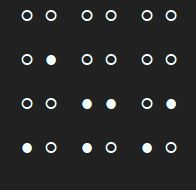/Python

# Python binary clock

During the Covid19 Quarantine I decided to exercise my mind a spend a few minutes coding a binary clock.

A binary clock is a clock that displays the time of day in a binary format. wikipedia.

A few minutes later and here is what I came up with.

``````import time
from datetime import datetime
from typing import List

def display_row() -> None:
current_time = datetime.now().time()
time_parts = [pad([int(i) for i in list(str(current_time.hour))]),

rows: List[int] = [8, 4, 2, 1]
for row in rows:
for part in time_parts:
display(part, row)
print(' ', end='')
print('')

while len(time_unit) < 2:
time_unit.insert(0, 0)
return time_unit

def display(time_unit: List[int], seed: int) -> None:
for index, value in enumerate(time_unit):
if value - seed >= 0:
time_unit[index] = value - seed
print('● ', end='')
else:
print('○ ', end='')

while True:
time.sleep(1)
# hack to move through pycharm run window
print('\n' * 80)
display_row()``````

Sample output for the time 14:32:12### Subscribe to Thulani S. Chivandikwa

Get the latest posts delivered right to your inbox#### Thulani S. Chivandikwa

husband, dad, .net developer, tech and tea lover